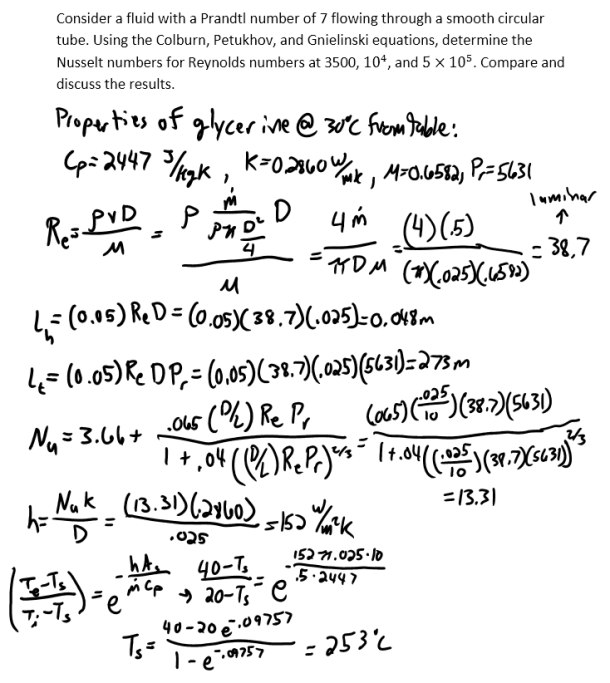heat and mass transfer problems engineering equations heat and mass transfer school homework engineering heat and mass transfer formulas heat and mass transfer solutions to heat and mass transfer problems full solution engineering problem solution heat and mass transfer math problems engineering equations heat and mass transfer school homework engineering solutions to heat and mass transfer formulas heat problems mass problem solutions to transfer problems full solution heat and mass transfer
heat and mass transfer problems engineering equations heat and mass transfer school homework engineering heat and mass transfer formulas heat and mass transfer solutions to heat and mass transfer problems full solution engineering problem solution heat and mass transfer math problems engineering equations heat and mass transfer school homework engineering solutions to heat and mass transfer formulas heat problems mass problem solutions to transfer problems full solution heat and mass transfer
Highalphabet Home Page heat and mass transfer problem solutions Heat and Mass Transfer Page
Consider a fluid with a Prandtl number of 7 flowing through a smooth circular tube. Using the Colburn, Petukhov, and Gnielinski equations, determine the Nusselt numbers for Reynolds numbers at 3500, 10^4, and 5×10^5. Compare and discuss the results.Consider a fluid with a Prandtl number of 7 flowing through a smooth circular tube. Using the Colburn, Petukhov, and Gnielinski equations, determine the Nusselt numbers for Reynolds numbers at 3500, 10^4, and 5×10^5. Compare and discuss the results.